# Painlevé-type equations

Jump to: navigation, search

Consider anth order homogeneous linear ordinary differential equation, either scalar: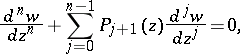or an-system:normalized such that the coefficient of the highest derivative is one. If the coefficients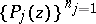are all analytic at a point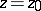in the complex plane, then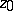is said to be a regular point of the ordinary differential equation and the equation haslinearly independent solutions in a neighbourhood of. Any singularities in the solutions to the ordinary differential equation must be located at the singularities of the coefficientsof the equation. These singularities are called fixed because they are determined by the coefficients in the equation. Their location is independent of the constants of integration. A prototypical situation is: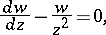whose general solution is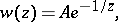being an arbitrary constant. The singularity in the solution is at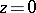, corresponding to the singularity in the coefficient: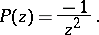Non-linear ordinary differential equations can have "internal" or "movable" singularities. The location of a movable singularity (cf. Movable singular point) depends on the constants of integration. A simple example iswhose general solution isA problem studied in the late 19th century and early 20th century involved classifying ordinary differential equations on the basis of the singularities they admit. A review of this work can be found in [a1]. Any singularity of a solution of an ordinary differential equation that is not a pole is called a critical point. This includes branch points and essential singular points (cf. Branch point: Essential singular point). In 1884 W.H.J. Fuchs showed that amongst the first-order equations of the form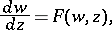whereis rational inand locally analytic in, the only equations without movable critical points are Riccati equations (cf. Riccati equation):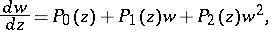which are linearizable to a second-order ordinary differential equation.

S. Kovalevskaya was awarded the Bordin Prize in 1888 for her remarkable discovery regarding the motion of a rigid body about a fixed point under the influence of gravity. Motivated by the work of Fuchs she looked for and found the choices of parameters for which the governing equations admit no movable critical points. She then showed that the equations can be explicitly integrated in these cases (an extensive discussion of Kovalevskaya's work is contained in [a2]).

Beginning near the turn of the century, P. Painlevé and his coworkers (notably B. Gambier) examined second-order equations of the form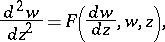whereis rational inand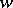and locally analytic in. They classified all such equations with the property that their solutions have no movable critical points. Today (1996) such equations are said to be of Painlevé type (or P type) or their solutions (or the equation) are said to possess the Painlevé property. It was shown by the Painlevé school that there are only some (depending on how one counts) 50 canonical equations whose solutions have no movable critical points. They showed that all of these equations may be reduced to an equation which is either linear or already solved (e.g. by an elliptic function) or to one of six "new" non-linear non-autonomous equations — the so-called six Painlevé transcendents, the first two of which are, in normalized form,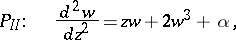withan arbitrary constant.

The question of which ordinary differential equations have solutions with the Painlevé property is natural to ask. This is particularly relevant given the connection between integrable non-linear evolution equations and Painlevé-type equations (see below). Unfortunately, a complete classification does not exist at higher order. However, an important discovery was made by J. Chazy (a discussion of Chazy's work can be found in [a3]). He showed that third-order non-linear ordinary differential equations of the form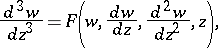whereis rational in,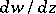,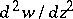and locally analytic in, could possess movable natural boundaries. The prototypical example is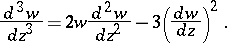## Painlevé property and integrable systems.

In their study of the long-time asymptotic structure of non-linear evolution equations solvable by the inverse scattering transform (IST; cf. Evolution equation), M.J. Ablowitz and A. Newell and Ablowitz and H. Segur (cf. [a4]) discovered that similarity solutions of the evolution equation played a significant role. The situation is best explained by an example. Consider the modified Korteweg–de Vries equation (mKdV)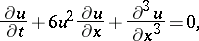which arises in many physical problems (e.g. plasma physics, fluid dynamics, non-linear optics). As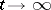, the solution decomposes into finite amplitude waves, called solitons (cf. Soliton), and a dispersive tail. The tail is dominated by the similarity solution of the modified Kortweg–de Vries equation: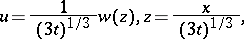where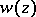satisfies:The latter equation integrates to the second Painlevé transcendent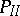discussed above.

In fact, Ablowitz and Segur showed that many of the well-known integrable non-linear wave equations solvable by the inverse scattering transform have similarity reductions which reduce to equations of Painlevé type. For example, a similarity solution of the Boussinesq equation reduces to the first Painlevé transcendent, and a similarity solution of the sine-Gordon equation can be transformed to the third Painlevé equation. Subsequently, researchers have shown that all of the classical second-order Painlevé equations (cf. also Painlevé equation) can be obtained as reductions of integrable partial differential equations (a large number of references may be found in [a3]).

The connections are much deeper than to the classical Painlevé equations. In fact, the integrable partial differential equations solvable by the inverse scattering transform have two general features that result. Firstly, their ordinary differential equation reductions have the Painlevé property, i.e. they do not have movable critical points. Although there is not a general proof of this statement available, nevertheless it can be proven that: 1) a subclass of solutions obtained via the linear integral equations of the inverse scattering transform satisfy this property (see [a4]); 2) once an ordinary differential equation is obtained there is a test, called the Painlevé test or, more generally, Painlevé analysis, which can be applied to establish that there is a full-dimensional family of solutions which have Laurent series representations in a neighbourhood of pole-type singularities (or sometimes more general singularities). This analysis can be generalized to apply, formally speaking, to partial differential equations (cf. Painlevé test). [a5], [a3].